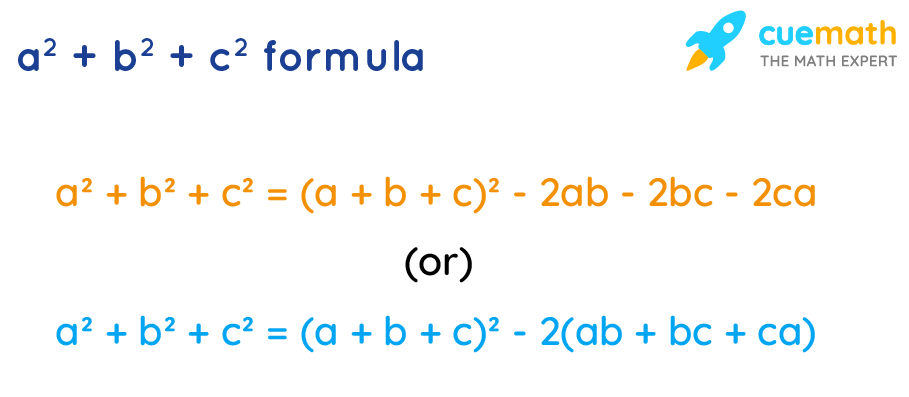# a^2 + b^2 + c^2 Formula

The a2 + b2 + c2 formula is used to find the sum of squares of three numbers without actually calculating the squares.

a2 + b2 + cformula is derived from the formula:

(a + b + c)2 = a2 + b2 + c2 + 2ab + 2bc + 2ca

Are you wondering how to derive this a^2 + b^2 + c^2 formula? We just multiply (a + b + c) by itself to get (a + b + c)2.

(a + b + c)2

= (a + b + c)(a + b + c)

= a+ ab + ac + ab + b+ bc + ca + bc + c2

= a2 + b2 + c2 + 2ab + 2bc + 2ca

## What Is a^2 + b^2 + c^2 Formula?

Subtracting 2ab + 2bc + 2ca from both sides of the above formula, the a2 + b2 + c2 formula is:## Solved Examples Using a^2 + b^2 + c^2 Formula

### Find the value of a2 + b2 + c2 if a + b + c = 10 and ab + bc + ca = -2.

Solution:

To find: a2 + b2 + c2.

Given that:

a + b + c = 10

ab + bc + ca = -2

Using the a2 + b2 + c2 formula,

a2 + b2 + c2 = (a + b + c)2 - 2(ab + bc + ca)

a2 + b2 + c2 = (10)2 - 2(-2) = 100 + 4 = 104

Answer: a2 + b2 + c2 = 104.

### Find the value of a2 + b2 + c2 if a + b + c = -3, 1/a + 1/b + 1/c = -2 and abc = 3.

Solution:

To find: a2 + b2 + c2.

Given that:

a + b + c = -3 ... (1)

1/a + 1/b + 1/c = -2 ... (2)

abc = 3 ... (3)

Multiplying (2) and (3),

\begin{align*} abc \left(\dfrac 1 a + \dfrac 1 b + \dfrac 1 c\right) &= (3)(-2)\\[0.2cm] bc + ca + ab &=-6 \end{align*}

Using the a2 + b2 + c2 formula,

a2 + b2 + c2 = (a + b + c)2 - 2(ab + bc + ca)

a2 + b2 + c2 = (-3)2 - 2(-6) = 9 + 12 = 21

Answer: a2 + b2 + c2 = 21.